# Quadratic equation + time - math problems

#### Number of problems found: 33

• Two trucksTwo trucks left cities A and B against each other and met after an hour. The first car came to B 27 minutes later than the second car to A. Calculate the car speed if the distance between cities A, B is 90 km.
• Work togetherMichal and Dorota would do the work together in 2.25 hours. If Dorota had to do it herself, it would have taken her 6 hours longer than Michal. Determine the time that Dorota would do the work herself and the time that Michal would do it himself.
• Blueberries 2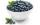Lenka and Martin collected eight liters of blueberries in two hours. How long will take to collect blueberries by Lenka itself, if we know that she collect all 2.5 hours less than Martin?
• Two pipes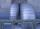One pipe fill one-fifth volume 20 minutes before by second one. The two pipes together will fill the tank in two hours. How long is will fill tank each pipe separately?
• Two masons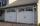Two masons built the garage together - it took 18 days. If they worked independently, the other would work 15 days more than the first. For how many days would build the garage each mason himhelp?
• Water reservoir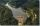The water reservoir is filled through one inlet 4 hours later than both together, then another inlet 9 hours later. For how long is filled by each separately?
• Copiers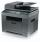The new copier copying a folder of papers 5 min. faster than the old. The operator used new, but out of toner and exchange took 5 min. In that time copied on the old. The whole work has been done for 9 min. How long would the work done only by old copier?
• Tourist Jirka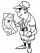Distance between the points A and B is 13.5 km. Jirka went from point A to point B unknown speed and for an unknown period of time. Back to the point A went slower by 3 km/h which means that went 20 minutes more. How long Jirka took the return journey?
• TrainsFrom station 130 km away started passenger train and after 2.2 hours after the express train, which travels 37 km an hour more. Express train finish journey 7 minutes early. Calculate the average speed of this two trains.
• AbyssThe stone fell into the abyss: 2 seconds after we heard hitting bottom. How deep is the abyss (neglecting air resistance)? (gravitational acceleration g = 9.81 m/s2 and the speed of sound in air v = 343 m/s)
• PoolIf water flows into the pool by two inlets, fill the whole for 19 hours. The first inlet filled pool 5 hour longer than the second. How long pool take to fill with two inlets separately?
• Wind drift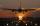The plane flies at 900 km/h, passing distance 1800 kilometers with the wind and once again against the wind for 4 h 1 min. What is the wind speed?
• Trolleybus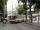Trolleybus line No. 206 measured 24 km. If the trolleybus goes faster by 5 km/h, the way there and back would is shorter by 33 minutes. Calculate the trolleybus speed and how much time it takes a return trip.

We apologize, but in this category are not a lot of examples.
Do you have an exciting math question or word problem that you can't solve? Ask a question or post a math problem, and we can try to solve it.

We will send a solution to your e-mail address. Solved examples are also published here. Please enter the e-mail correctly and check whether you don't have a full mailbox.

Looking for help with calculating roots of a quadratic equation? Do you want to convert time units like minutes to seconds? Quadratic Equations Problems. Time - math problems.